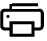# Sine,Cosine,Tangent,Cotangent,Arcsin,Arccos,Arctan Tables Generator# Radians Degree Table GeneratorOne of most powerfull table generator that you can generate any number of Trigonometry Tables instantly.

Here is the explanation about the input parameters

Start Value - Starting Value (It will generate the next 100 values from Start value)

Step Value - What will be the increment value after Start value.

Example: Your start value is 10, and Step Value is 1 ,

Then Sin(10 + 1) = Sin(11) = ? , Sin(11 + 1) = Sin(12) = ? , Sin(12 + 1) = Sin(13) = ? , Sin(13 + 1) = Sin(14) = ? and so on.

You can give the step value of both decimal and whole numbers.

Precision - Rounding off the output values

## Arctangent Formulas

The arctangent function is the inverse function of y = tan(x).

arctan(y) = tan-1(y) = x+

## Arccos Formulas

The arccosine function is the inverse function of cos(x).

arccos(x) = cos-1(x)

## Arcsine Formulas

The arcsine function is the inverse function of y = sin(x).

arcsin(y) = sin-1(y) = x + 2##Related Math Calculators

Online math number calculation, formulas , Online Algebra calculation, formulas , Matrix calculation, formulas , Digital calculation, Statistical calculation